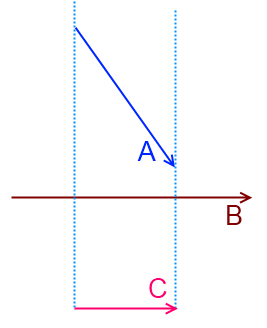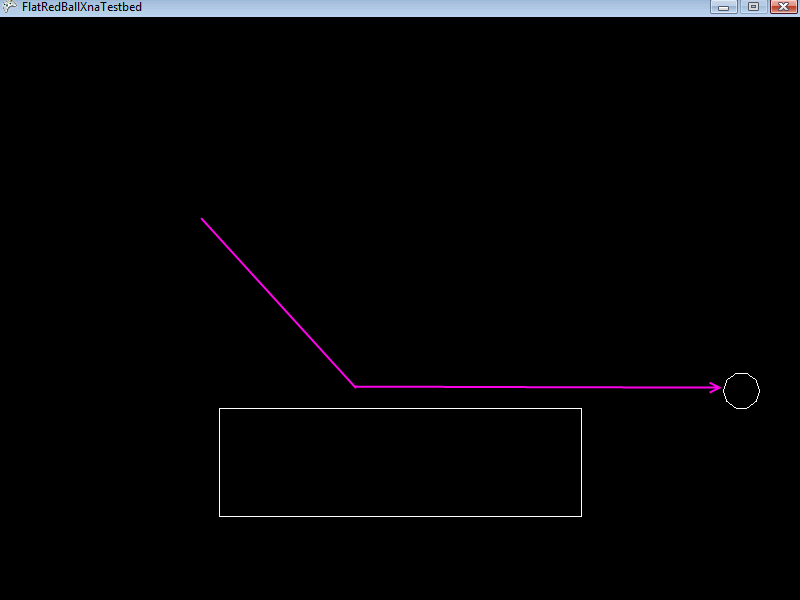FlatRedBall.Math.Geometry.Polygon.ProjectParentVelocityOnLastMoveCollisionTangent

Introduction

The ProjectParentVelocityOnLastMoveCollisionTangent method is a very powerful and useful method for creating realistic collision. This method can be used in many types of games to help reduce “tunneling” – that is, objects hopping through other objects because of high speeds.

What does this actually mean?

The ProjectParentVelocityOnLastMoveCollisionTangent property is a very long property name which takes a little bit of understanding. Let’s break it down.

To explain what this property means, we’ll start from the back and go to the front. First, we’ll start with:

OnLastMoveCollision

This phrase indicates that the property that we are investigating will give us information about the last time a “Move” collision was called. In other words, the last time CollideAgainstMove was called. That’s all!

OnLastMoveCollision + Tangent

Next, we’ll add the Tangent property. In math, a tangent is a line which moves parallel (in the same direction) as a surface. The following image shows a blue line which is a tangent on the yellow circleThe phrase “on last move collision tangent” means that we are going to do something with the line (or Vector in more precise terms) that is tangential to the point where the collision happened the last time CollideAgainstMove was called.

Project…Velocity + OnLastMoveCollisionTangent

Next we’ll look at the phrase Project Velocity. The “…” appears in the title because we’re going to explain why the word “Parent” appears after this section. A projection in Linear Algebra is the operation done between two Vectors where one Vector is modified so that it is parallel to the second vector, and its length is set to be its length along the second vector. This is a difficult concept to explain in words, so observe the following picture:In this picture Vector A is projected onto Vector B, resulting in Vector 3. Notice that the result will always be parallel to the projected-on Vector.

Assuming there is no bouncing, friction, or energy loss from the collision, this projection realistically represents the behavior of a moving object as it collides with another object. In other words, projecting the velocity on the tangent of the last collision will make the object appear to collide with the object and keep moving in a realistic manner after the collision.

Project + Parent + VelocityOnLastMoveCollisionTangent

Finally we introduce the word “Parent”. The reason Parent appears in this method name is because most shapes are never used alone, but rather are used as children of another object (usually Entities). Therefore, the shape shouldn’t modify its own Velocity, but rather the Velocity of its parent.

CollideAgainstBounce

This method is only used in more complex situations. You can usually accomplish the exact same behavior by simply calling CollideAgainstBounce with an elasticity of 0.

Method Signature

ProjectParentVelocityOnLastMoveCollisionTangent()
ProjectParentVelocityOnLastMoveCollisionTangent(float minimumVectorLengthSquared)

minimumVectorLengthSquared

The minimumVectorLengthSquared argument is an argument that can control whether velocity modifications should be performed. Shapes which have the ProjectParentVelocityOnLastMoveCollisionTangent method store the vector that they were moved along in the last CollideAgainstMove call. This property is the called LastMoveCollisionReposition. The minimumVectorLengthSquared is a value which is compared against the square of the length of LastMoveCollisionReposition. This can be used to prevent the projection of velocity.

Most of the time this is not needed, and calling the no-argument version of ProjectParentVelocityOnLastMoveCollisionTangent uses a default value of 0 for minimumVectorLengthSquared. This argument can be used in very rare cases where large numbers can result in precision loss.

Code example

The following code creates two Polygons. The smaller polygon will move down and to the right, then slide along the larger polygon. Once the smaller polygon reaches the end of the larger polygon, it will continue to move to the right. In other words, it will lose its “downward” component of its velocity vector.

using FlatRedBall.Math.Geometry;

Add the following at class scope:

Polygon mLargePolygon;
Polygon mSmallPolygon;

Add the following to Initialize after initializing FlatRedBall:

mLargePolygon = Polygon.CreateRectangle(10, 3);
mLargePolygon.Y = -8;
mSmallPolygon = Polygon.CreateEquilateral(10, 1, 0);
mSmallPolygon.X = -4;
mSmallPolygon.XVelocity = 2;
mSmallPolygon.YVelocity = -2;

if (mSmallPolygon.CollideAgainstMove(mLargePolygon, 0, 1))
{
mSmallPolygon.ProjectParentVelocityOnLastMoveCollisionTangent();
}Things to try: If you remove the call to

mSmallPolygon.ProjectParentVelocityOnLastMoveCollisionTangent();

you will notice that the moving Polygon will continue to move downward after reaching the end of the larger Polygon. Give it a shot to see the difference.

Creating a Basic Platforming Engine

ProjectParentVelocityOnLastMoveCollisionTangent is useful both for realistic physics, as well as semi-realistic physics as found in many platformers (such as Super Mario Bros.)

The following is a block of code which can serve as an example for how to handle collision in a platformer:

// Assume that myPolygon and polygonList are valid references to a Polygon and
// a PositionedObjectList<Polygon>, respectively
bool onGround = false;
foreach (Polygon polygon in polygonList)
{
// The 0 is the relative mass of myPolygon and 1 is the relative
// mass of the argument polygon.  In this case, the ground is infinitely
// more massive than myPolygon.
if (myPolygon.CollideAgainstMove(polygon, 0, 1))
{
// Adjust the velocity of myPolygon
myPolygon.ProjectParentVelocityOnLastMoveCollisionTangent();
// If myPolygon was moved upward then it is resting on the ground
// Keep in mind this could be a sharp slope as well.
onGround|= myPolygon.LastMoveCollisionReposition.Y > 0;
}
}